# polarplot

Plot line in polar coordinates

•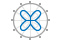## Syntax

``polarplot(theta,rho)``
``polarplot(theta,rho,LineSpec)``
``polarplot(theta1,rho1,...,thetaN,rhoN)``
``polarplot(theta1,rho1,LineSpec1,...,thetaN,rhoN,LineSpecN)``
``polarplot(rho)``
``polarplot(rho,LineSpec)``
``polarplot(Z)``
``polarplot(Z,LineSpec)``
``polarplot(tbl,thetavar,rhovar)``
``polarplot(tbl,rhovar)``
``polarplot(pax,___)``
``polarplot(___,Name,Value)``
``p = polarplot(___)``

## Description

### Vector and Matrix Data

example

````polarplot(theta,rho)` plots a line in polar coordinates, with `theta` indicating the angle in radians and `rho` indicating the radius value for each point. The inputs must be vectors of equal length or matrices of equal size. If the inputs are matrices, then `polarplot` plots columns of `rho` versus columns of `theta`. Alternatively, one of the inputs can be a vector and the other a matrix as long as the vector is the same length as one dimension of the matrix.```

example

````polarplot(theta,rho,LineSpec)` sets the line style, marker symbol, and color for the line.```
````polarplot(theta1,rho1,...,thetaN,rhoN)` plots multiple `rho,theta` pairs.```
````polarplot(theta1,rho1,LineSpec1,...,thetaN,rhoN,LineSpecN)` specifies the line style, marker symbol, and color for each line.```

example

````polarplot(rho)` plots the radius values in `rho` at evenly spaced angles between 0 and 2π.```
````polarplot(rho,LineSpec)` sets the line style, marker symbol, and color for the line.```

example

````polarplot(Z)` plots the complex values in `Z`.```
````polarplot(Z,LineSpec)` sets the line style, marker symbol, and color for the line.```

### Table Data

example

````polarplot(tbl,thetavar,rhovar)` plots the variables `thetavar` and `rhovar` from the table `tbl`. To plot one data set, specify one variable for `thetavar` and one variable for `rhovar`. To plot multiple data sets, specify multiple variables for `thetavar`, `rhovar`, or both. If both arguments specify multiple variables, they must specify the same number of variables. (since R2022a)```
````polarplot(tbl,rhovar)` plots the radius values in `rhovar` at evenly spaced angles between 0 and 2π. Timetables are not supported for this syntax. (since R2022a)```

````polarplot(pax,___)` uses the `PolarAxes` object specified by `pax`, instead of the current axes.```
````polarplot(___,Name,Value)` specifies properties of the chart line using one or more `Name,Value` pair arguments. The property settings apply to all the lines. You cannot specify different property values for different lines using `Name,Value` pairs.```

example

````p = polarplot(___)` returns one or more chart line objects. Use `p` to set properties of a specific chart line object after it is created. For a list of properties, see Line Properties.```

## Examples

collapse all

Plot a line in polar coordinates.

```theta = 0:0.01:2*pi; rho = sin(2*theta).*cos(2*theta); polarplot(theta,rho)```Before R2022a, polar axes do not include degree symbols by default. To add them, get the polar axes using `pax = gca`. Then modify the tick labels using `pax.ThetaTickLabel = string(pax.ThetaTickLabel) + char(176)`.

Create the data to plot.

```theta = linspace(0,360,50); rho = 0.005*theta/10;```

Convert the values in `theta` from degrees to radians. Then, plot the data in polar coordinates.

```theta_radians = deg2rad(theta); polarplot(theta_radians,rho)```Plot two lines in polar coordinates. Use a dashed line for the second line.

```theta = linspace(0,6*pi); rho1 = theta/10; polarplot(theta,rho1) rho2 = theta/12; hold on polarplot(theta,rho2,'--') hold off```Specify only the radius values, without specifying the angle values. `polarplot` plots the radius values at equally spaced angles that span from 0 to $2\pi$. Display a circle marker at each data point.

```rho = 10:5:70; polarplot(rho,'-o')```Create a polar plot using negative radius values. By default, `polarplot` reflects negative values through the origin.

```theta = linspace(0,2*pi); rho = sin(theta); polarplot(theta,rho)```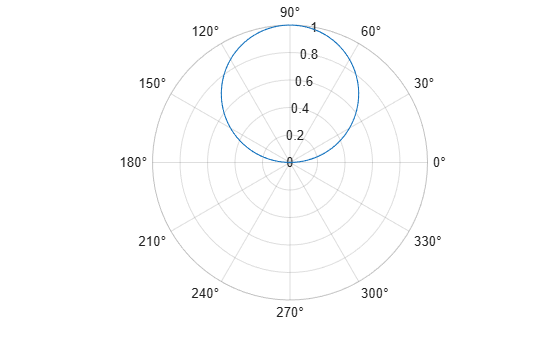Change the limits of the r-axis so it ranges from -1 to 1.

`rlim([-1 1])`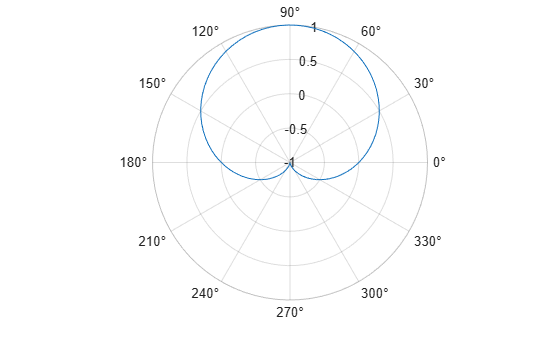Create a polar plot using a red line with circle markers.

```theta = linspace(0,2*pi,25); rho = 2*theta; polarplot(theta,rho,'r-o')```Create a polar plot and return the chart line object.

```theta = linspace(0,2*pi,25); rho = 2*theta; p = polarplot(theta,rho);```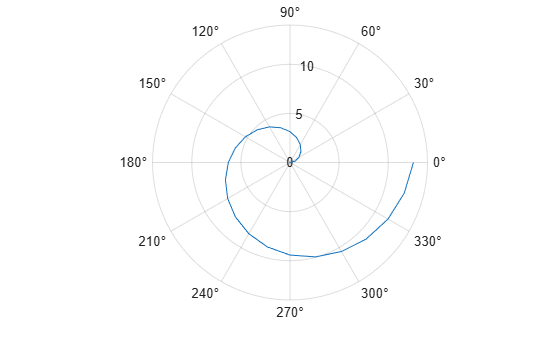Change the line color and width and add markers.

```p.Color = 'magenta'; p.Marker = 'square'; p.MarkerSize = 8;```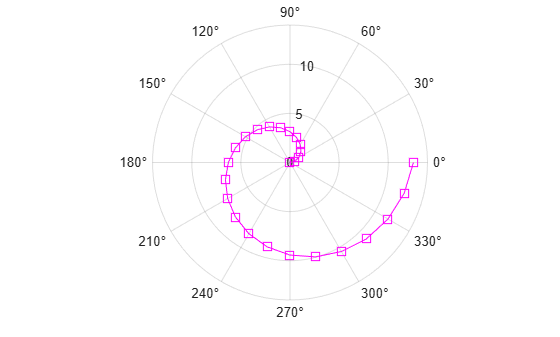Plot complex values in polar coordinates. Display markers at each point without a line connecting them.

```Z = [2+3i 2 -1+4i 3-4i 5+2i -4-2i -2+3i -2 -3i 3i-2i]; polarplot(Z,'*')```A convenient way to plot data from a table is to pass the table to the `polarplot` function and specify the variables to plot.

Create a table with two variables. Then display the first three rows of the table.

```Angle = linspace(0,3*pi,50)'; Radius = (1:50)'; tbl = table(Angle,Radius); head(tbl,3)```
``` Angle Radius _______ ______ 0 1 0.19234 2 0.38468 3 ```

Plot the `Angle` and `Radius` variables. Return the `Line` object as `p`.

`p = polarplot(tbl,"Angle","Radius");`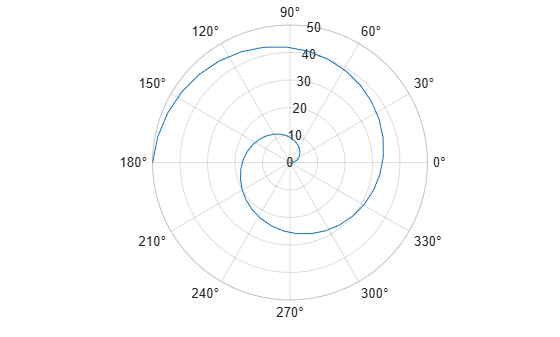To modify aspects of the line, set the `LineStyle`, `Color`, and `Marker` properties on the `Line` object. For example, change the line to a red dotted line with circle markers.

```p.LineStyle = ":"; p.Color = "red"; p.Marker = "o";```Create a table with three variables. Then display the first three rows in the table.

```Angle = linspace(0,3*pi,50)'; Radius1 = (1:50)'; Radius2 = Radius1/2; tbl = table(Angle,Radius1,Radius2); head(tbl,3)```
``` Angle Radius1 Radius2 _______ _______ _______ 0 1 0.5 0.19234 2 1 0.38468 3 1.5 ```

Plot the `Radius1` and `Radius2` variables against the `Angle` variable. Add a legend. Notice that the legend labels match the variable names.

```polarplot(tbl,"Angle",["Radius1" "Radius2"]) legend```## Input Arguments

collapse all

Angle values, specified as a vector or matrix. Specify the values in radians. To convert data from degrees to radians, use `deg2rad`.

To change the limits of the theta-axis, use `thetalim`.

Example: `[0 pi/2 pi 3*pi/2 2*pi]`

Radius values, specified as a vector or matrix. By default, negative values are reflected through 0. A point is reflected by taking the absolute value of its radius, and adding 180 degrees to its angle.

To change the limits of the r-axis, use `rlim`.

Example: `[1 2 3 4 5]`

Complex values, specified as a vector or matrix where each element is of the form `rho*`ei*theta, or `x+iy`, where:

• `rho = sqrt(x^2+y^2)`

• `theta = atan(y/x)`

Example: `[1+2i 3+4i 3i]`

Line style, marker, and color, specified as a string scalar or character vector containing symbols. The symbols can appear in any order. You do not need to specify all three characteristics (line style, marker, and color). For example, if you omit the line style and specify the marker, then the plot shows only the marker and no line.

Example: `"--or"` is a red dashed line with circle markers.

Line StyleDescriptionResulting Line
`"-"`Solid line`"--"`Dashed line`":"`Dotted line`"-."`Dash-dotted lineMarkerDescriptionResulting Marker
`"o"`Circle`"+"`Plus sign`"*"`Asterisk`"."`Point`"x"`Cross`"_"`Horizontal line`"|"`Vertical line`"square"`Square`"diamond"`Diamond`"^"`Upward-pointing triangle`"v"`Downward-pointing triangle`">"`Right-pointing triangle`"<"`Left-pointing triangle`"pentagram"`Pentagram`"hexagram"`HexagramColor NameShort NameRGB TripletAppearance
`"red"``"r"``[1 0 0]``"green"``"g"``[0 1 0]``"blue"``"b"``[0 0 1]``"cyan"` `"c"``[0 1 1]``"magenta"``"m"``[1 0 1]``"yellow"``"y"``[1 1 0]``"black"``"k"``[0 0 0]``"white"``"w"``[1 1 1]`Source table containing the data to plot, specified as a table or a timetable.

Table variables containing the theta values, specified using one of the indexing schemes from the table.

Indexing SchemeExamples

Variable names:

• `"A"` or `'A'` — A variable named `A`

• `["A","B"]` or `{'A','B'}` — Two variables named `A` and `B`

• `"Var"+digitsPattern(1)` — Variables named `"Var"` followed by a single digit

Variable index:

• An index number that refers to the location of a variable in the table.

• A vector of numbers.

• A logical vector. Typically, this vector is the same length as the number of variables, but you can omit trailing `0` or `false` values.

• `3` — The third variable from the table

• `[2 3]` — The second and third variables from the table

• `[false false true]` — The third variable

Variable type:

• `vartype("categorical")` — All the variables containing categorical values

The table variables you specify can contain any real numeric data type. If `thetavar` and `rhovar` both specify multiple variables, the number of variables must be the same.

Example: `polarplot(tbl,["th1","th2"],"rho")` specifies the table variables named `th1` and `th2` for the theta-coordinates.

Example: `polarplot(tbl,2,"rho")` specifies the second variable for the theta-coordinates.

Example: `polarplot(tbl,vartype("numeric"),"rho")` specifies all numeric variables for the theta-coordinates.

Table variables containing the rho values, specified using one of the indexing schemes from the table.

Indexing SchemeExamples

Variable names:

• `"A"` or `'A'` — A variable named `A`

• `["A","B"]` or `{'A','B'}` — Two variables named `A` and `B`

• `"Var"+digitsPattern(1)` — Variables named `"Var"` followed by a single digit

Variable index:

• An index number that refers to the location of a variable in the table.

• A vector of numbers.

• A logical vector. Typically, this vector is the same length as the number of variables, but you can omit trailing `0` or `false` values.

• `3` — The third variable from the table

• `[2 3]` — The second and third variables from the table

• `[false false true]` — The third variable

Variable type:

• `vartype("categorical")` — All the variables containing categorical values

The table variables you specify can contain any real numeric data type. If `thetavar` and `rhovar` both specify multiple variables, the number of variables must be the same.

Example: `polarplot(tbl,"theta",["rho1","rho2"])` specifies the table variables named `rho1` and `rho2` for the radius values.

Example: `polarplot(tbl,"theta",2)` specifies the second variable for the radius values.

Example: `polarplot(tbl,"theta",vartype("numeric"))` specifies all numeric variables for the radius values.

`PolarAxes` object. You can modify the appearance and behavior of a `PolarAxes` object by setting its properties. For a list of properties, see PolarAxes Properties.

### Name-Value Arguments

Specify optional pairs of arguments as `Name1=Value1,...,NameN=ValueN`, where `Name` is the argument name and `Value` is the corresponding value. Name-value arguments must appear after other arguments, but the order of the pairs does not matter.

Before R2021a, use commas to separate each name and value, and enclose `Name` in quotes.

Example: `'LineWidth',3`

`Name,Value` pair settings apply to all the lines plotted. You cannot specify different `Name,Value` pairs for each line using this syntax. Instead, return the chart line objects and use dot notation to set the properties for each line.

The properties listed here are only a subset. For a full list, see Line Properties.

Line color, specified as an RGB triplet, a hexadecimal color code, a color name, or a short name.

For a custom color, specify an RGB triplet or a hexadecimal color code.

• An RGB triplet is a three-element row vector whose elements specify the intensities of the red, green, and blue components of the color. The intensities must be in the range `[0,1]`, for example, ```[0.4 0.6 0.7]```.

• A hexadecimal color code is a string scalar or character vector that starts with a hash symbol (`#`) followed by three or six hexadecimal digits, which can range from `0` to `F`. The values are not case sensitive. Therefore, the color codes `"#FF8800"`, `"#ff8800"`, `"#F80"`, and `"#f80"` are equivalent.

Alternatively, you can specify some common colors by name. This table lists the named color options, the equivalent RGB triplets, and hexadecimal color codes.

Color NameShort NameRGB TripletHexadecimal Color CodeAppearance
`"red"``"r"``[1 0 0]``"#FF0000"``"green"``"g"``[0 1 0]``"#00FF00"``"blue"``"b"``[0 0 1]``"#0000FF"``"cyan"` `"c"``[0 1 1]``"#00FFFF"``"magenta"``"m"``[1 0 1]``"#FF00FF"``"yellow"``"y"``[1 1 0]``"#FFFF00"``"black"``"k"``[0 0 0]``"#000000"``"white"``"w"``[1 1 1]``"#FFFFFF"``"none"`Not applicableNot applicableNot applicableNo color

Here are the RGB triplets and hexadecimal color codes for the default colors MATLAB® uses in many types of plots.

`[0 0.4470 0.7410]``"#0072BD"``[0.8500 0.3250 0.0980]``"#D95319"``[0.9290 0.6940 0.1250]``"#EDB120"``[0.4940 0.1840 0.5560]``"#7E2F8E"``[0.4660 0.6740 0.1880]``"#77AC30"``[0.3010 0.7450 0.9330]``"#4DBEEE"``[0.6350 0.0780 0.1840]``"#A2142F"`Line style, specified as one of the options listed in this table.

Line StyleDescriptionResulting Line
`"-"`Solid line`"--"`Dashed line`":"`Dotted line`"-."`Dash-dotted line`"none"`No lineNo line

Line width, specified as a positive value in points, where 1 point = 1/72 of an inch. If the line has markers, then the line width also affects the marker edges.

The line width cannot be thinner than the width of a pixel. If you set the line width to a value that is less than the width of a pixel on your system, the line displays as one pixel wide.

Marker symbol, specified as one of the values listed in this table. By default, the object does not display markers. Specifying a marker symbol adds markers at each data point or vertex.

MarkerDescriptionResulting Marker
`"o"`Circle`"+"`Plus sign`"*"`Asterisk`"."`Point`"x"`Cross`"_"`Horizontal line`"|"`Vertical line`"square"`Square`"diamond"`Diamond`"^"`Upward-pointing triangle`"v"`Downward-pointing triangle`">"`Right-pointing triangle`"<"`Left-pointing triangle`"pentagram"`Pentagram`"hexagram"`Hexagram`"none"`No markersNot applicable

Marker size, specified as a positive value in points, where 1 point = 1/72 of an inch.

Marker fill color, specified as `"auto"`, an RGB triplet, a hexadecimal color code, a color name, or a short name. The `"auto"` option uses the same color as the `Color` property of the parent axes. If you specify `"auto"` and the axes plot box is invisible, the marker fill color is the color of the figure.

For a custom color, specify an RGB triplet or a hexadecimal color code.

• An RGB triplet is a three-element row vector whose elements specify the intensities of the red, green, and blue components of the color. The intensities must be in the range `[0,1]`, for example, ```[0.4 0.6 0.7]```.

• A hexadecimal color code is a string scalar or character vector that starts with a hash symbol (`#`) followed by three or six hexadecimal digits, which can range from `0` to `F`. The values are not case sensitive. Therefore, the color codes `"#FF8800"`, `"#ff8800"`, `"#F80"`, and `"#f80"` are equivalent.

Alternatively, you can specify some common colors by name. This table lists the named color options, the equivalent RGB triplets, and hexadecimal color codes.

Color NameShort NameRGB TripletHexadecimal Color CodeAppearance
`"red"``"r"``[1 0 0]``"#FF0000"``"green"``"g"``[0 1 0]``"#00FF00"``"blue"``"b"``[0 0 1]``"#0000FF"``"cyan"` `"c"``[0 1 1]``"#00FFFF"``"magenta"``"m"``[1 0 1]``"#FF00FF"``"yellow"``"y"``[1 1 0]``"#FFFF00"``"black"``"k"``[0 0 0]``"#000000"``"white"``"w"``[1 1 1]``"#FFFFFF"``"none"`Not applicableNot applicableNot applicableNo color

Here are the RGB triplets and hexadecimal color codes for the default colors MATLAB uses in many types of plots.

`[0 0.4470 0.7410]``"#0072BD"``[0.8500 0.3250 0.0980]``"#D95319"``[0.9290 0.6940 0.1250]``"#EDB120"``[0.4940 0.1840 0.5560]``"#7E2F8E"``[0.4660 0.6740 0.1880]``"#77AC30"``[0.3010 0.7450 0.9330]``"#4DBEEE"``[0.6350 0.0780 0.1840]``"#A2142F"`## Tips

• To convert data from degrees to radians, use `deg2rad`. To convert data from radians to degrees, use `rad2deg`.

• You can modify polar axes properties to customize the chart. For a list of properties, see PolarAxes Properties.

• To plot additional data in the polar axes, use the ```hold on``` command. However, you cannot plot data that requires Cartesian axes in a polar chart.

## Version History

Introduced in R2016a

expand all